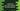# How to sort array objects in JavaScript by value property# Introduction :

We can easily sort an array of strings or an array of numbers in Javascript. But, how can we sort an array of objects? For example, if you have an array of student objects with name, age, and marks for each, what is the easiest way to sort these students based on their marks or name or age? In this tutorial, we will learn to sort such arrays. In real-world, this is the most commonly faced problem and Javascript provides one simple way to solve it.

## Array sort method in Javascript :

Javascript array has one method called sort(). This method can be used to sort all elements of an array. It is defined as below :

``````array.sort([function])
``````

### Arguments :

It takes one single argument, i.e. one function. This is an optional value. Based on this function, it sorts the array elements. If we don’t pass the optional function argument, it sorts the elements in ascending order.

sort() function sorts the array elements in place. Based on the return value of the function, the sorting is done.

• For arguments a and b, if the return value is less than 0, the sorting will place a before b.
• For arguments a and b, if the return value is greater than 0, the sorting will place b before a.
• For arguments a and b, if the return value is equal to 0, the order will remain the same.

### Sorting an array of objects :

We can sort an array of objects using the sort method with a function. Let’s create one array of Student objects :

``````    let students = [];

students = {name : "Alex", marks : 20, age : 12};
students = {name : "Bob", marks : 30, age : 13};
students = {name : "Charlie", marks : 28, age : 14};
``````

To sort this array based on different properties, we can write different functions and pass it to the sort method like below :

``````    function sortAgeDescending(s1, s2) {
return s2.age - s1.age;
}

function sortMarksAscending(m1, m2) {
return m1.marks - m2.marks;
}
let students = [];

students = { name: "Alex", marks: 20, age: 12 };
students = { name: "Bob", marks: 30, age: 13 };
students = { name: "Charlie", marks: 28, age: 14 };

console.log(students.sort(sortAgeDescending))
console.log('\n')
console.log(students.sort(sortMarksAscending))
``````

Here, we have created two different functions sortAgeDescending and sortMarksAscending. Both take two arguments i.e. two student objects. sortAgeDescending returns one positive value if the age of the second argument is greater than the first argument and negative value if the age of the first argument is greater than the second argument. So, it will sort all elements in ascending age.

Similarly, sortMarksAscending returns positive value if the marks of the first argument are more than the second argument and negative value if the marks of the second argument are more than the first argument.

We can pass any of these function to the sort method to sort the array based on a different property.

### Conclusion :

Javascript array sort() method provides an easy way to sort the array elements. We can sort simple elements like string, integer or any complex objects like we have seen above. Try to run the example shown above and drop one comment below if you have any queries.